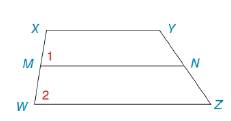Chapter 2.3, Problem 22E### Elementary Geometry for College St...

6th Edition
Daniel C. Alexander + 1 other
ISBN: 9781285195698

#### Solutions

Chapter
Section### Elementary Geometry for College St...

6th Edition
Daniel C. Alexander + 1 other
ISBN: 9781285195698
Textbook Problem
1 views

# In Exercise 19 to 22 complete the proof. Given: X Y ¯ ∥ Y Z ¯ ∠ 1 ≅ ∠ 2 Prove: M N ¯ ∥ X Y ¯To determine

To find:

The complete proof of the given statement.

Explanation

Given:

The given statement is,

XY¯WZ¯ and 12.

Figure (1)

Theorem:

(1). If two lines are cut by a transversal so that two corresponding angles are congruent, then these lines are parallel.

(2). If two lines are each parallel to a third line, then these lines are parallel to each other.

Approach:

The given statement is,

XY¯WZ¯ and 12.

1 and 2 are congruent corresponding angles.

Therefore, MN¯ is parallel to WZ¯.

MN¯WZ¯ and XY¯WZ¯.

Lines MN¯ and XY¯ each are parallel to line WZ¯.

Therefore, MN¯ is parallel to line XY¯.

The complete proof of the given statement is shown in the following table,

 Proof Statements Reasons 1

### Still sussing out bartleby?

Check out a sample textbook solution.

See a sample solution

#### The Solution to Your Study Problems

Bartleby provides explanations to thousands of textbook problems written by our experts, many with advanced degrees!

Get Started

#### In Problems 29-36, find the particular solution to each differential equation.

Mathematical Applications for the Management, Life, and Social Sciences

#### Evaluate the integral. 16. z10zdz

Single Variable Calculus: Early Transcendentals

#### Sometimes, Always, or Never: (ax) = ax.

Study Guide for Stewart's Single Variable Calculus: Early Transcendentals, 8th